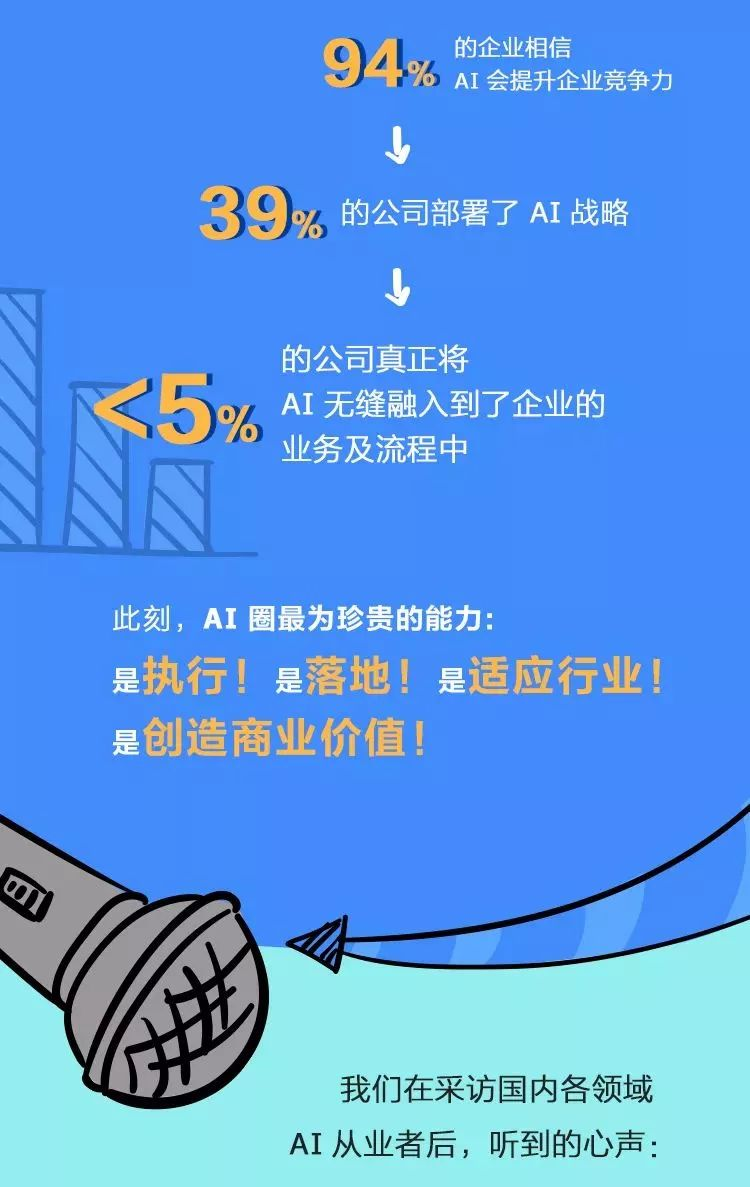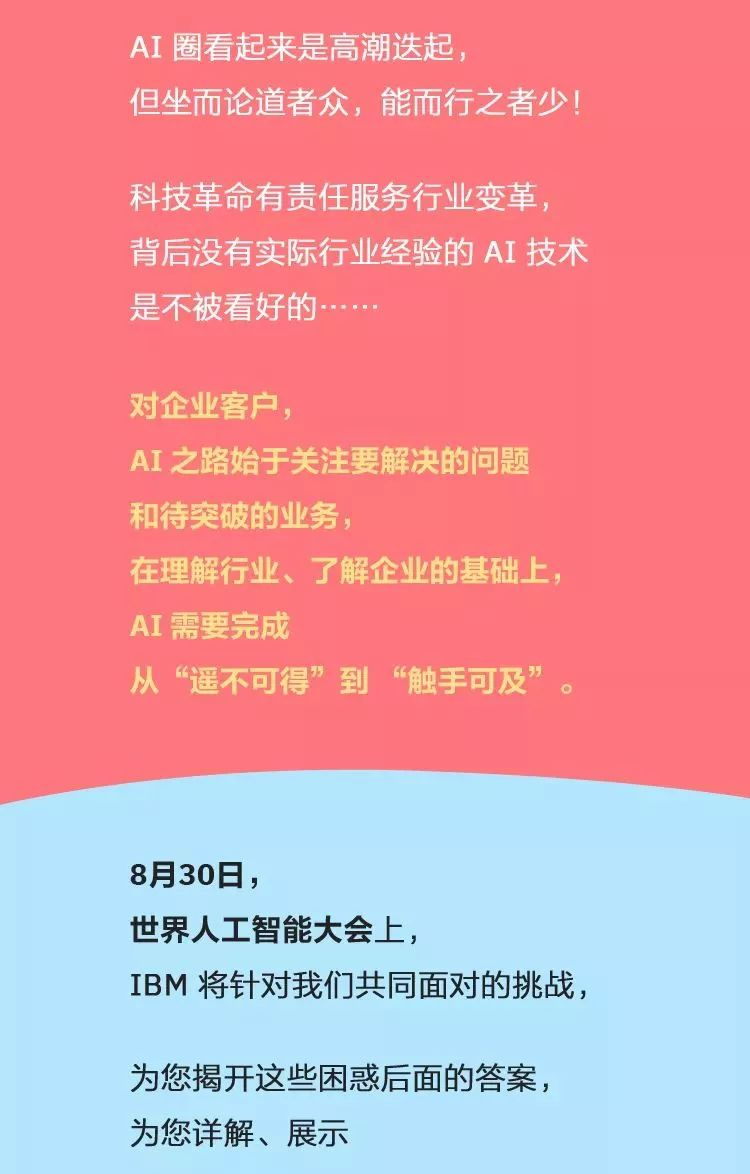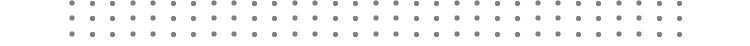## 比吐槽？没人说得过我们做AI的

8 月 19 日 51CTO博客0+0+

### 相关内容0+0+0+0+0+0+0+0+0+0+0+0+0+0+0+0+1+0+0+1+0+0+0+0+0+0+0+0+1+0+
Top